Important Questions: Fractions and Decimals

# Important Questions: Fractions and Decimals

Test Description

## 20 Questions MCQ Test Mathematics (Maths) Class 7 | Important Questions: Fractions and Decimals

Important Questions: Fractions and Decimals for Class 7 2022 is part of Mathematics (Maths) Class 7 preparation. The Important Questions: Fractions and Decimals questions and answers have been prepared according to the Class 7 exam syllabus.The Important Questions: Fractions and Decimals MCQs are made for Class 7 2022 Exam. Find important definitions, questions, notes, meanings, examples, exercises, MCQs and online tests for Important Questions: Fractions and Decimals below.
Solutions of Important Questions: Fractions and Decimals questions in English are available as part of our Mathematics (Maths) Class 7 for Class 7 & Important Questions: Fractions and Decimals solutions in Hindi for Mathematics (Maths) Class 7 course. Download more important topics, notes, lectures and mock test series for Class 7 Exam by signing up for free. Attempt Important Questions: Fractions and Decimals | 20 questions in 20 minutes | Mock test for Class 7 preparation | Free important questions MCQ to study Mathematics (Maths) Class 7 for Class 7 Exam | Download free PDF with solutions
 1 Crore+ students have signed up on EduRev. Have you?
Important Questions: Fractions and Decimals - Question 1

### Which of the following is true with respect to 9/16 and 13/5?

Detailed Solution for Important Questions: Fractions and Decimals - Question 1

L.C.M. is 16×5 =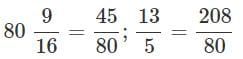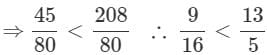Important Questions: Fractions and Decimals - Question 2

### Which of the following is the improper fraction form of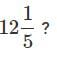Detailed Solution for Important Questions: Fractions and Decimals - Question 2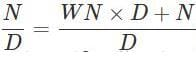where W is the whole number, N is the numerator and D is the denominator. So,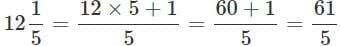Important Questions: Fractions and Decimals - Question 3

### What is the sum of 3/4,7/6  and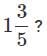Detailed Solution for Important Questions: Fractions and Decimals - Question 3

To find the sum of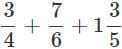Find the L.C.M. of the denominators and then add them. L.C.M. of 4, 5 and 6 is 60.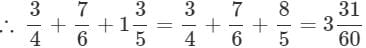Important Questions: Fractions and Decimals - Question 4

What is the difference 13/15 and 11/35 ?

Detailed Solution for Important Questions: Fractions and Decimals - Question 4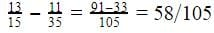Important Questions: Fractions and Decimals - Question 5

Which of the following is a proper fraction?

Important Questions: Fractions and Decimals - Question 6

What is the product of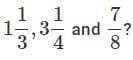Detailed Solution for Important Questions: Fractions and Decimals - Question 6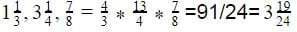Important Questions: Fractions and Decimals - Question 7

Guru reads 3/5 of a book. He finds that there are still 80 pages left to be read. What is the total number of pages in the book?

Detailed Solution for Important Questions: Fractions and Decimals - Question 7

No. pages left =(1−3/5)×  total pages
∴Total number of pages = 80× 5/22 = 200

Important Questions: Fractions and Decimals - Question 8

What is 1/7 of 49 litres?

Detailed Solution for Important Questions: Fractions and Decimals - Question 8

1/7× 49  litres and 7 litres.

Important Questions: Fractions and Decimals - Question 9

Indian cricket team won 4 more matches than it lost with New Zealand. If it won 3/5 of its matches, how many matches did India play?

Detailed Solution for Important Questions: Fractions and Decimals - Question 9

Let the number of matches lost hex. The number of matches won = x+4.
Total matches played  = x+x+4
We have,  x+4 = 3/5 (2x+4) ⇒ x = 8
∴  Total matches played = 20

Important Questions: Fractions and Decimals - Question 10

What is the product of a fractional number and its reciprocal?

Detailed Solution for Important Questions: Fractions and Decimals - Question 10

The product of a fraction and its reciprocal is always 1.

In the case of a whole number, think of it as having a denominator of 1: The reciprocal of 5 is 1/5.

multiplying 5 and 1/5 gives 1.

Every number has a reciprocal except for 0.

Important Questions: Fractions and Decimals - Question 11

What should be added to 21/27  to make it 26/27 ?

Important Questions: Fractions and Decimals - Question 12

What are fractions with different denominators called?

Important Questions: Fractions and Decimals - Question 13

What is the equivalent fraction of 8/11 having the numerator 40?

Important Questions: Fractions and Decimals - Question 14

What do you call the fractions which have one as the numerator?

Important Questions: Fractions and Decimals - Question 15

In which of the following fractions is the numerator greater than the denominator?

Important Questions: Fractions and Decimals - Question 16

1/9 of a number is 5.What is the number?

Detailed Solution for Important Questions: Fractions and Decimals - Question 16

Let 'x' be the number. 1/9  of  x = 5 ⇒ x = 5×9 = 45

Important Questions: Fractions and Decimals - Question 17

Given that P/q = s/t, which of these is true?

Detailed Solution for Important Questions: Fractions and Decimals - Question 17

P/q = s/t ⇒ pt = sq (By cross multiplication.)

Important Questions: Fractions and Decimals - Question 18

What should be added to 3 to get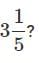Detailed Solution for Important Questions: Fractions and Decimals - Question 18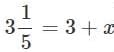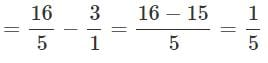Important Questions: Fractions and Decimals - Question 19

If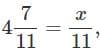what is the value of x?

Detailed Solution for Important Questions: Fractions and Decimals - Question 19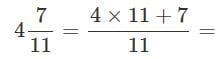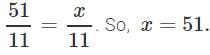Important Questions: Fractions and Decimals - Question 20

What is the lowest form of 12/30 ?

Detailed Solution for Important Questions: Fractions and Decimals - Question 20

12/30 = 2/5 is the required lowest form.

## Mathematics (Maths) Class 7

168 videos|252 docs|45 tests
 Use Code STAYHOME200 and get INR 200 additional OFF Use Coupon Code
Information about Important Questions: Fractions and Decimals Page
In this test you can find the Exam questions for Important Questions: Fractions and Decimals solved & explained in the simplest way possible. Besides giving Questions and answers for Important Questions: Fractions and Decimals, EduRev gives you an ample number of Online tests for practice

## Mathematics (Maths) Class 7

168 videos|252 docs|45 tests